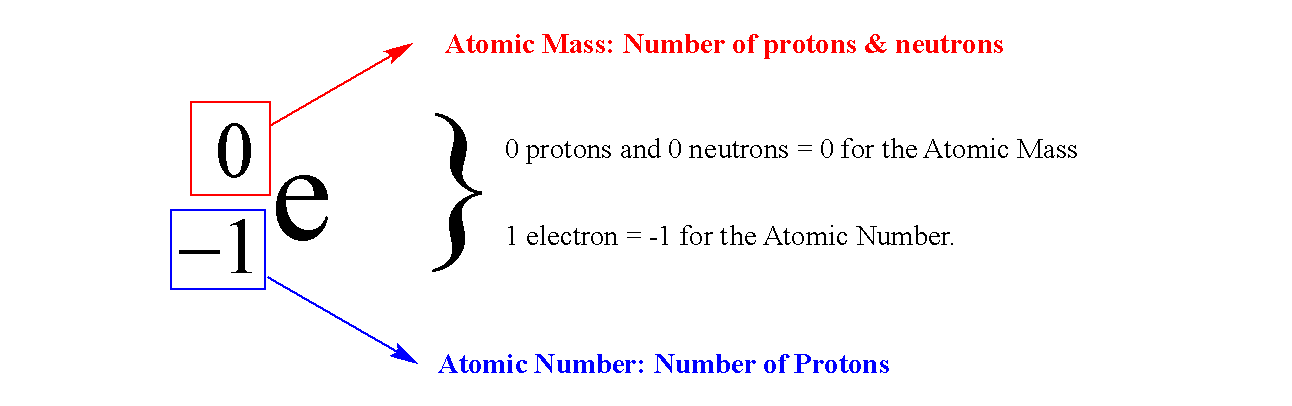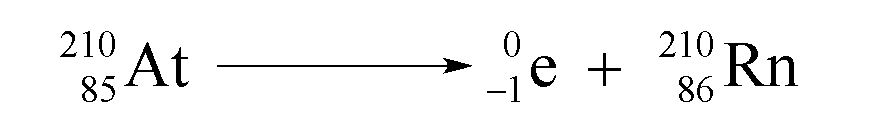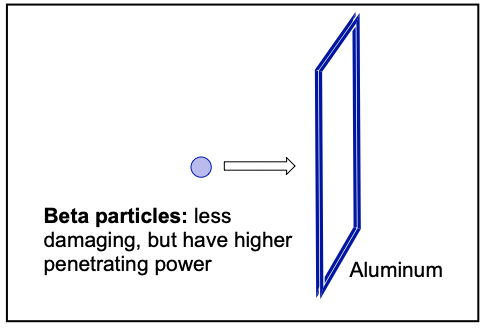Clutch Prep is now a part of Pearson
Ch.11 Nuclear ChemistryWorksheetSee all chapters

# Beta Decay

See all sections
Sections
Alpha Decay
Beta Decay
Gamma Emission
Electron Capture
Positron Emission

A beta decay or beta emission occurs when an unstable nucleus ejects a beta particle to create a new element.

###### Understanding Beta Decay

A beta particle has no atomic mass and is represented by an electron.Concept #1: Understanding Beta Decay or Emission

Transcript

Hey guys in this new video, we’re going to take a look at Beta Decay, now we’re going to say that Beta decay occurs when an unstable nucleus somehow emits a(n) electron. Now we’re going to say beta particle can be represented by e for the electron. Electron here we going to say is much smaller than the other 2 sub atomic particles, so the atomic mass can just be understood as 0e and here we going to say atomic number is -1 0e because the atomic number is basically the number of protons since this is an electron, we’re going to say that it’s the opposite of a proton which is 1 so an electron is -1.
Now we’re going to say here Beta decay can be represented when we emit a beta particle so for example if we had Mercury 201 and remember that 201 means its atomic mass, so let’s say we had Mercury 201 if we look on our periodic table, Mercury has an atomic number of 80. We’re going to emit a beta particle 1 0e, now remember you’re going to say your atomic masses have to be equal to each other on both sides of the arrow and your atomic numbers as well. Here the electron has no mass so the new element is going to have a mass of 201 and then here we have to be very careful here this is -1 so - 1 + what gives me 80? Well the answer would have to be 81 because 81 - 1 gives me the 80 that I had originally. So just remember that. So here would be Tl so this would be example of beta decay or a beta emission.Concept #2: The Beta Particle

Transcript

Now we’re going to say in terms of size, we know that the alpha particles are the largest radioactive particle, so we’re going to say beta particles therefore are smaller than alpha particles. Now because they’re smaller in size they’re going to be less damaging if we ingest them. So here, they’re going to have a lower ionizing power but the problem here is because they’re smaller they’re able to better penetrate into our skin. So here they’re going to have more penetrating power and so the stopping sheet of metal or large block of wood to make sure beta particle does not enter inside our bodies.

Beta particles are smaller in size, and therefore have more penetrating power. Luckily, they are less radioactively damaging because of their lower ionizing power.Example #1: Write balanced nuclear equations for each of the following beta emissions.

a) Magnesium (Mg) – 25

b) Ruthenium (Ru) – 102

Transcript

So, here we have to write the balanced nuclear equations for each of the following beta emissions, again, beta decay, beta emissions means that the beta particle will be a product, if they had said beta capture or beta absorption then it would be a reacted.
Example 1: So here were starting out with Magnesium 25, 25Mg so that’s the atomic mass.
On our periodic table Magnesium has an atomic number of 12.
1225Mg  -1 0e +1325Al
It’s going to emit a beta particle, -1 0e , so our new element that’s being created would still have the same atomic mass, and then here -1 + what number gives me 12? It’d have to be 13, because 13 - 1 gives me the 12 I started out with initially. So this element would be Aluminum (Al).
Now Ruthenium (Ru) 102, were going to say if we look on our periodic table Ruthenium has an atomic number of 44.
44102Ru  -1 0e +45105Rh
Again were going to emit a beta particle so this number stays 102 and this number it would have to be 45. So goes up to Rh.
Ok, so those are the examples of beta decays or beta emissions.

Example #2: Pb – 208 is formed from Th -232. How many alpha and beta decays have occurred?

a. 6, 2      b. 6, 6      c. 6, 4      d. 4, 6      e. 8, 2